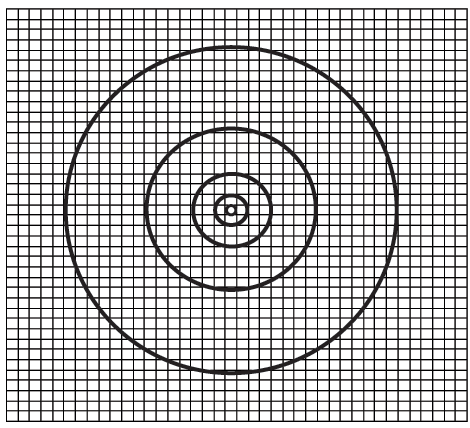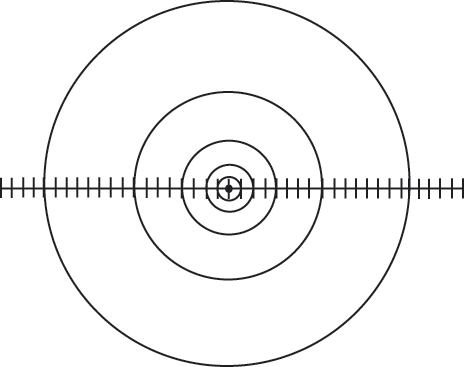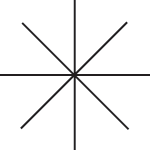HandsOn 7 - Circle Measurement of Dimension

One method we have been using to measure fractal dimension is the method of covering. There are other methods for measuring dimension. One that is useful for objects with dimension less than or equal to two involves measuring the total area of the object present inside circles of different radii. For a surface in a plane, the area increases as the square of the radius: A =R2. The power 2 of R tells us-again!-that a disk is 2-dimensional.

Check results of the formula A =R2 by counting the number of cells inside circles of different radii in the Figure. For each circle, count all the cells inside that circle, not just those between that circle and the next smaller circle. Enter your results in a copy of Table 2.5.Figure 2.7: Count the cells inside each circle to discover that the surface of the figure is 2-dimensional.

Table 2.5: For use with Figure 2.7.

 r (radius) N (count) 0.5 1.5 3.5 7.5 15.5

Plot the results from your copy of Table 2.5 on a log-log graph, the radius scale along the horizontal axis and N along the vertical axis. From the measurement of the slope, calculate the dimension of the circular surface in Figure 2.7.

Now measure the dimension of the line in Figure using a similar procedure. First, count the number N of short line segments inside each circle of radius r. Enter the results in a copy of Table 2.8. Second, plot N versus r on a log-log graph and measure the slope. What is the value of the dimension of the line?Figure 2.8: Count the number of line segments inside each circle to discover again that a line is 1-dimensional.

Table 2.6: For use with Figure 2.8.
 r (radius) N (count) 1 2 4 8 16

 Q2.11: Suppose the line in Figure 2.8 were not not straight, but curved. What dimension would it have, according to the circle method? Cut a piece of string to the length of the line in Figure 2.8, lay it down curved on Figure 2.8 and check its dimension with the circle method.

Q2.12: What is the dimension of this pattern? Is this a fractal? What would be the best method for calculating its dimension ?Previous: HandsOn 6 - 1-, 2-, & 3-Dimensions

Next: 2.3 - Mathematical Fractals